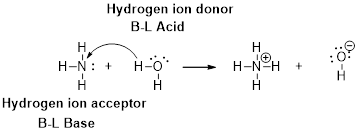Chapter 16, Problem 9PS

Chapter
Section
Textbook Problem

An aqueous solution has a pH of 3.75. What is the hydronium ion concentration of the solution? Is it acidic or basic?

Interpretation Introduction

Interpretation:

The hydronium ion concentration of the solution has to be determined and the acid or basic character has to be explained.

Concept introduction:

An acid was defined as a substance whose turns blue litmus to red, water solution tastes sour and neutralizes the bases.

A base was defined as a substance whose turns red litmus to blue, aqueous solution tasted bitter and neutralizes acids.

Bronsted –Lowry definitions:

A Bronsted –Lowry acid is a proton donor, it donates a hydrogen ion, H+

A Bronsted-Lowry base is a proton acceptor, it accepts a hydrogen ion H+Bronsted –Lowry conjugated acid-Base pairs:

When an acid is dissolved in water, the acid (HA) donates a proton to water to form a new acid (conjugated acid) and a new base (conjugated base).The pair of an Acid –Base differs by a proton called conjugated Acid-Base pair.

Explanation

From the given the pH of the solution =3.75

Let’s calculate the hydronium ion concentration:

pH = -log[H+]3.75 = -log[H3O+][H3O+] = 10-3

Still sussing out bartleby?

Check out a sample textbook solution.

See a sample solution

The Solution to Your Study Problems

Bartleby provides explanations to thousands of textbook problems written by our experts, many with advanced degrees!

Get Started